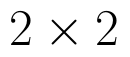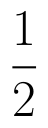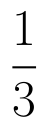Select Page

# 12 Science CBSE Solutions for MCQ Maths Relations and Functions in English

12 Science CBSE Solutions for MCQ Maths Relations and Functions in English to enable students to get Solutions in a narrative video format for the specific question.

Expert Teacher provides 12 Science CBSE Solutions for MCQ Maths Relations and Functions through Video Solutions in English language. This video solution will be useful for students to understand how to write an answer in exam in order to score more marks. This teacher uses a narrative style for a question from Relations and Functions not only to explain the proper method of answering question, but deriving right answer too.

Please find the question below and view the Solution in a narrative video format.

Question:

Solution Video in English:

You can select video Solutions from other languages also. Please check Solutions in ( Hindi )

## Similar Questions from CBSE, 12th Science, Maths, Relations and Functions

Question 2 : Ifis defined by, write f(f(x)). (View Answer Video)

Question 3 : Ifandare onto, thenis: (View Answer Video)

Question 4 : Letbe defined as. Choose the correct answer. (View Answer Video)

Question 5 : Functionsare defined respectively, by, find. (View Answer Video)

### Matrices

Question 1 : Compute:. (View Answer Video)

Question 2 : If for any squarematrix A,then write the value of |A|. (View Answer Video)

Question 3 : Find the inverse of the matrix,. (View Answer Video)

Question 4 : Find the value of x, if. (View Answer Video)

Question 5 : Find the value of X, ifand. (View Answer Video)

### Probability

Question 1 : In a hockey match, both teams A and B scored same number of goals up to the end of the game, so to decide the winner, the referee asked both the captains to throw a die alternately and decided that the team, whose captain gets a six first, will be declared the winner. If the captain of the team A was asked to start, find their respective probabilities of winning the match and state whether the decision of the referee was fair or not.      (View Answer Video)

Question 2 : An experiment succeeds thrice as often as it fails. Find the probability that in the next trials, there will be atleast 3 successes.    (View Answer Video)

Question 3 : A die is thrown 6 times. If 'getting an odd number' is a 'success', what is the probability of obtaining:
exactly 5 successes?

Question 4 : Assume that the chances of a patient having a heart attack are 40%. Assume that a meditation and yoga course reduces the risk of heart attack by 30% and the prescription of certain drugs and certain restrictions reduces the risk by 25%. At a time, a patient choses only one of the two options with equal probabilities. After going through one of the two options, the patient is selected at random who is suffering from a heart attack. Find the probability that the patient followed a course of meditation and yoga. Write the value referred here.      (View Answer Video)

Question 5 :  Probability of solving specific problem independently by A and B areandrespectively. If both try to solve the problem, independently, then find the probability that the problem is solved.
Question 1 : Find :(View Answer Video)
Question 2 : Find. (View Answer Video)
Question 3 : Prove that :(View Answer Video)
Question 4 : Find the integral of the function. (View Answer Video)
Question 5 : Evaluate :(View Answer Video)